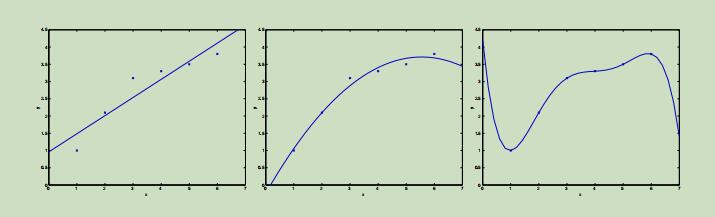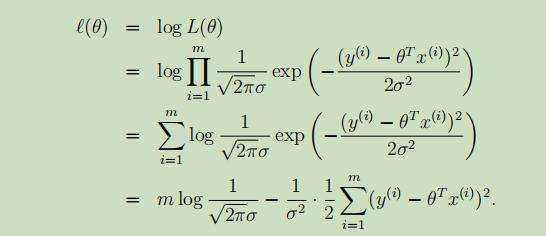# 吴恩达Stanford机器学习公开课（三）笔记

## Lecture 3 -Weighted Least Squares. Logistic Regression.

Posted by Yunlongs on January 21, 2019

# Lecture 3 -Weighted Least Squares. Logistic Regression.

## 1. 局部加权线性回归（Locally weighted linear regression）## 概率层面的解释# 分类和回归

## 2.逻辑回归（logistic regression）

### 2.1损失函数

y的取值只能为1或0，显而易见该损失函数是凸函数。

$\theta = argmin_\theta J(\theta) = argmin_\theta -\frac {1}{m} \sum _{i=1}^m \lbrace ylog\hat y+(1-y)log(1-\hat y) \rbrace$

## 3.逻辑回归-神经网络模式

• You get X
• You compute $A = \sigma(w^T X + b) = (a^{(1)}, a^{(2)}, …, a^{(m-1)}, a^{(m)})$
• You calculate the cost function: $J = -\frac{1}{m}\sum_{i=1}^{m}y^{(i)}\log(a^{(i)})+(1-y^{(i)})\log(1-a^{(i)})$

## 4.感知器算法（perceptron learning algorithm）